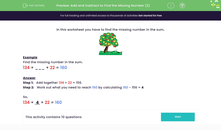# Add and Subtract to Find the Missing Number (2)

In this worksheet, students have to find the missing number in the sum by using addition and then subtraction.Key stage:  KS 2

Curriculum topic:   Number: Addition and Subtraction

Curriculum subtopic:   Solve Add/Subtract Problems to 1000

Difficulty level:#### Worksheet Overview

In this worksheet you have to find the missing number in the sum.Example

Find the missing number in the sum.

134 + ___ + 22 = 160

Step 1:   Add together 134 + 22 = 156.

Step 2:   Work out what you need to reach 160 by calculating 160 - 156 = 4

So,

134 +  4  + 22 = 160

### What is EdPlace?

We're your National Curriculum aligned online education content provider helping each child succeed in English, maths and science from year 1 to GCSE. With an EdPlace account you’ll be able to track and measure progress, helping each child achieve their best. We build confidence and attainment by personalising each child’s learning at a level that suits them.

Get started